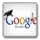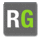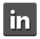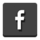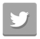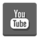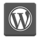You are in:Home/Publications/“Propagation of boundary of inhomogeneous heat conduction equation” accepted to Journal of Applied Mathematics and Computation.

Prof. Reda Gamal Abd El –Rahman Khaled :: Publications:

 Title: “Propagation of boundary of inhomogeneous heat conduction equation” accepted to Journal of Applied Mathematics and Computation. Authors: Reda G. Abd El -Rahman Year: 2003 Keywords: Moving boundary condition; Symmetry method Journal: Not Available Volume: 141, Issue: 2–3, 5 Pages: 231–239 Publisher: Not Available Local/International: Local Paper Link: Not Available Full paper Not Available Supplementary materials Not Available
 Abstract: We consider the problem of determining analytically the exact solutions of the heat conduction equation in an inhomogeneous medium, described by the diffusion equation ∂tT(x,t)=r1−s∂r(k(r)rs−1∂rT(r,t)) with a position-dependent thermal diffusivity K(r). The unsteady one-dimensional heat conduction equation is transformed into an ordinary differential equation called Kummer’s equation unifiedly in the linear, cylindrical and spherical coordinate systems. Kummer’s equation is solved in terms of the confluent hypergeometric functions. These solutions exist on the conditions that boundaries move with their positions proportional to some functions of time. Progress has been made in this direction by introducing similarity variables and transformations.